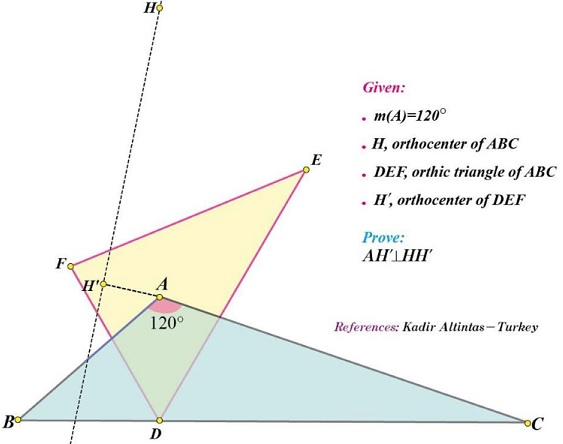# Orthic Triangle in a Triangle with a 120 degrees angle

### What Might This Be About?

13 February 2017, Created with GeoGebra

### Source### Problem### Remark

It's a well known property that three vertices of a triangle and its orthocenter form what is called the orthocentric system: the triangle formed by any three of the four points has the fourth point as its orthocenter. From this it follows that all four triangles share the same orthic triangle and, therefore, the orthocenter of the orthic triangle is one and the same for all four. If we consider just two pairs of triangle/orthocenter, e.g., $\{\Delta ABC, H\}\,$ and $\{\Delta HBC, A\},\,$ then, $A\,$ and $H\,$ simply swap their roles but not their locations, so the relation $HH'\perp AH',\,$ if it holds for one pair, necessarily holds for the other also.

Further, simple angle chasing shows that if $\angle BAC=120^{\circ},\,$ then $\angle BHC=60^{\circ}.\,$ This informs us that if the statement above holds for a triangle with a $120^{\circ}\,$ angle it also holds for triangles with a $60^{\circ},\,$ and vice versa.

### Acknowledgment

The above problem by Hadir Altintas (Turkey) has been posted at the CutTheKnotMath facebook page by Leo Giugiuc (Romania), with Takis Chronopoulos' English translation from the Greek of the solution by Stathis Koutras (Greece).